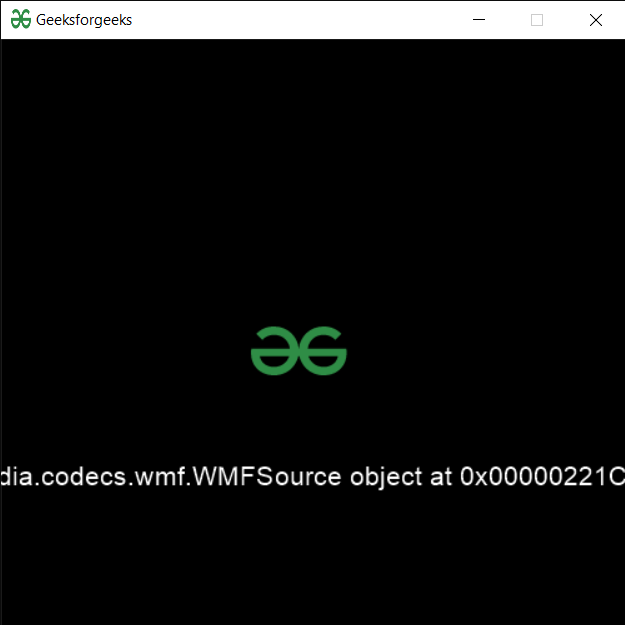Related Articles
PYGLET – Loading Media
• Last Updated : 24 Sep, 2020

In this article we will see how we can load a media file in PYGLET module in python. Pyglet is easy to use but powerful library for developing visually rich GUI applications like games, multimedia etc. A window is a “heavyweight” object occupying operating system resources. Windows may appear as floating regions or can be set to fill an entire screen (fullscreen). In order to load a file i.e resource we use resource module of pyglet. This module allows applications to specify a search path for resources. Relative paths are taken to be relative to the application’s __main__ module. Media is basically sound of video resource files

We can create a window object with the help of command given below

```# creating a window
window = pyglet.window.Window(width, height, title)
```

In order to do this we use `media` method with the pyglet.resource

Syntax : resource.media(file_name)

Argument : It takes string i.e file name as argument

Return : It returns media.Source object

Below is the implementation

 `# importing pyglet module ``import` `pyglet ``import` `pyglet.window.key as key``   ` `# width of window ``width ``=` `500``   ` `# height of window ``height ``=` `500``   ` `# caption i.e title of the window ``title ``=` `"Geeksforgeeks"``   ` `# creating a window ``window ``=` `pyglet.window.Window(width, height, title) ``   ` `# text  ``text ``=` `"Welcome to GeeksforGeeks"``  ` `# creating label with following proeprties``# font = cooper``# position = 250, 150``# anchor position = center``label ``=` `pyglet.text.Label(text, ``                          ``font_name ``=``'Cooper'``, ``                          ``font_size ``=` `16``, ``                          ``x ``=` `250``,  ``                          ``y ``=` `150``, ``                          ``anchor_x ``=``'center'``,  ``                          ``anchor_y ``=``'center'``)`` ` ` ` `# creating a batch ``batch ``=` `pyglet.graphics.Batch()`` ` `# loading geeksforgeeks image``image ``=` `pyglet.image.load(``'gfg.png'``)`` ` ` ` `# creating sprite object``# it is instance of an image displayed on-screen``sprite ``=` `pyglet.sprite.Sprite(image, x ``=` `200``, y ``=` `230``)``   ` `# on draw event ``@window``.event ``def` `on_draw(): ``       ` `    ``# clear the window ``    ``window.clear() ``       ` `    ``# draw the label``    ``label.draw() ``     ` `    ``# draw the image on screen``    ``sprite.draw()``       ` `# key press event     ``@window``.event ``def` `on_key_press(symbol, modifier): ``   ` `    ``# key "C" get press ``    ``if` `symbol ``=``=` `key.C: ``         ` `        ``# printng the message``        ``print``(``"Key : C is pressed"``)``         ` `# image for icon ``img ``=` `image ``=` `pyglet.resource.image(``"gfg.png"``) `` ` `# setting image as icon ``window.set_icon(img) `` ` `# loading media file``value ``=` `pyglet.resource.media(``"media.mp4"``)`` ` `# setting text  of label``label.text ``=` `str``(value)`` ` `# start running the application ``pyglet.app.run() `

Output :Attention geek! Strengthen your foundations with the Python Programming Foundation Course and learn the basics.

To begin with, your interview preparations Enhance your Data Structures concepts with the Python DS Course.

My Personal Notes arrow_drop_up